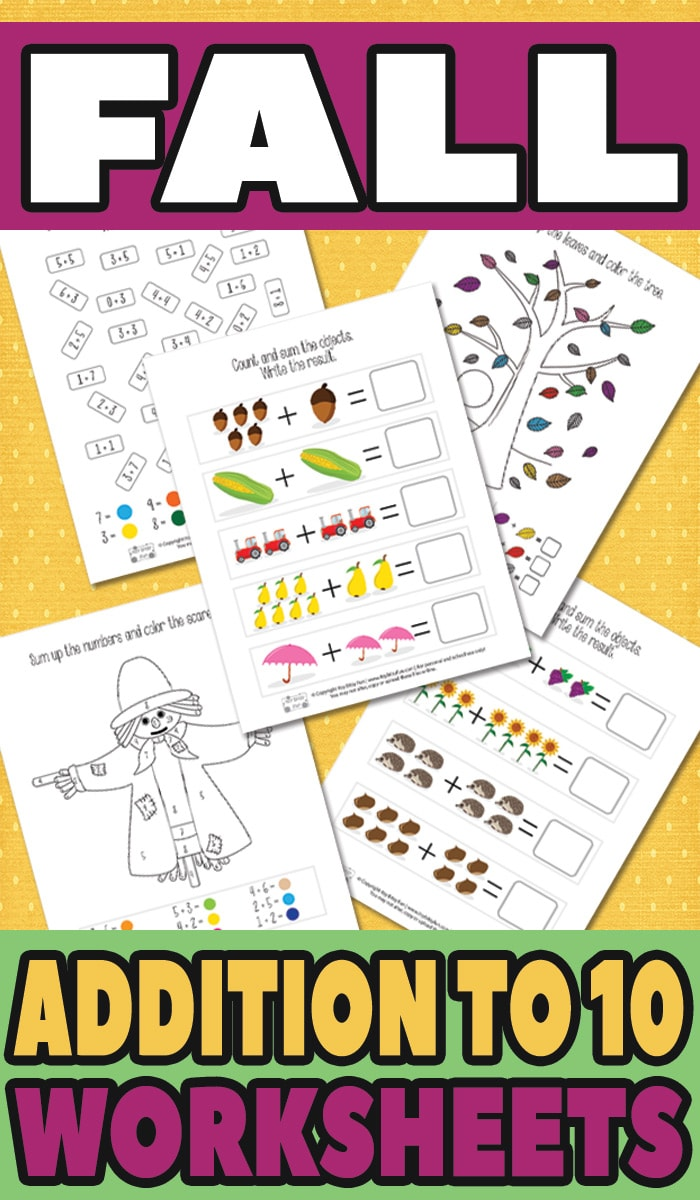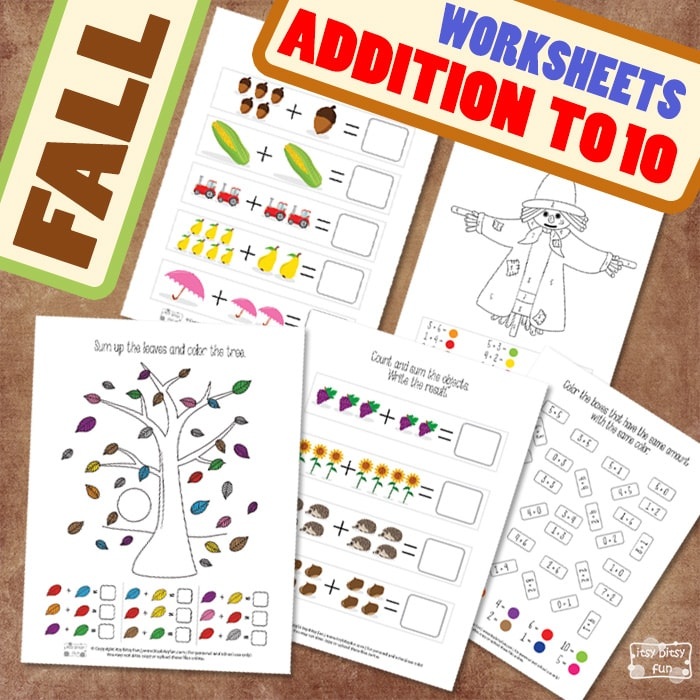# Fall Addition Worksheets to 10

Need to strengthen counting and addition skills? This time we’ve got a fun Fall Addition Worksheets for your young ones to practice on.

Math worksheets always come in handy when kids are trying to learn new ways of thinking when it comes to adding numbers.## Free Printable Fall Addition Worksheets

These free fall-themed addition to 10 worksheets are a perfect way to learn about how to add numbers.

On the first two pages, your kids will have to sum up the given objects and write down the total in the box at the end of each row.

The next page is a color by number exercise. Young mathematics need to add the numbers from the box in the bottom and figure out their sum to color the big scarecrow.

This exercise is great because they need to remember the sum and then color the area with the given color. Of course, they can write the sum into the colored circles to help themselves when coloring the image.

Continue with a fun page of counting and adding the tree’s leaves. There are 9 different colors of leaves that are falling down from a tree.  In this exercise, kids need to carefully count the leaves (that have the same color) to solve the addition equations.

On the last page, you will get another coloring exercise. Learn how different numbers (addends)  give the same sum and color those equations in the same color. Working with colors is great for recognizing the same amounts in equations and other math problems.

So, let’s have some fun math time and print out the worksheets!